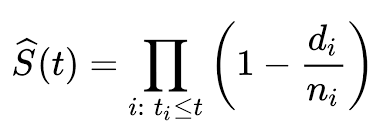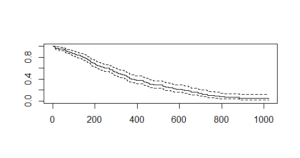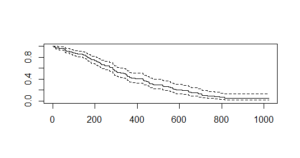Open In App

# Survival Analysis in R

Survival analysis in R Programming Language deals with the prediction of events at a specified time. It deals with the occurrence of an interesting event within a specified time and failure of it produces censored observations i.e incomplete observations.

## Survival analysis in R Programming Language

Biological sciences are the most important application of survival analysis in which we can predict the time for organisms eg. when they will multiply to sizes etc.

### Methods used to do survival analysis:

There are two methods that can be used to perform survival analysis in R programming language:

• Kaplan-Meier method
• Cox Proportional hazard model

## Kaplan-Meier Method

The Kaplan-Meier method is used in survival distribution using the Kaplan-Meier estimator for truncated or censored data. It’s a non-parametric statistic that allows us to estimate the survival function and thus not based on underlying probability distribution. The Kaplan–Meier estimates are based on the number of patients (each patient as a row of data) from the total number of patients who survive for a certain time after treatment. (which is the event).

We represent the Kaplan–Meier function by the formula:Here S(t) represents the probability that life is longer than t with ti(At least one event happened), di represents the number of events(e.g. deaths) that happened in time ti and ni represents the number of individuals who survived up to time ti.

Example:

We will use the Survival package for the analysis. Using Lung dataset preloaded in survival package which contains data of 228 patients with advanced lung cancer from North Central cancer treatment group based on 10 features. The dataset contains missing values so, missing value treatment is presumed to be done at your side before the building model.

## R

 `# Installing package``install.packages``(``"survival"``)` `# Loading package``library``(survival)` `# Dataset information``?lung` `# Fitting the survival model``Survival_Function = ``survfit``(``Surv``(lung\$time,``                                 ``lung\$status == 2)~1)``Survival_Function` `# Plotting the function``plot``(Survival_Function)`

Here, we are interested in “time” and “status” as they play an important role in the analysis. Time represents the survival time of patients. Since patients survive, we will consider their status as dead or non-dead(censored).

The Surv() function takes two times and status as input and creates an object which serves as the input of survfir() function. We pass ~1 in survfit() function to ensure that we are telling the function to fit the model on basis of the survival object and have an interrupt.

survfit() creates survival curves and prints the number of values, number of events(people suffering from cancer), the median time and 95% confidence interval. The plot gives the following output:Here, the x-axis specifies “Number of days” and the y-axis specifies the “probability of survival“. The dashed lines are upper confidence interval and lower confidence interval.

We also have the confidence interval which shows the margin of error expected i.e In days of surviving 200 days, upper confidence interval reaches 0.76 or 76% and then goes down to 0.60 or 60%

## Cox proportional hazard model

It is a regression modeling that measures the instantaneous risk of deaths and is bit more difficult to illustrate than the Kaplan-Meier estimator. It consists of hazard function h(t) which describes the probability of event or hazard h(e.g. survival) up to a particular time t. Hazard function considers covariates(independent variables in regression) to compare the survival of patient groups.
It does not assume an underlying probability distribution but it assumes that the hazards of the patient groups we compare are constant over time and because of this it is known as “Proportional hazard model“.

Example:

We will use the Survival package for the analysis. Using Lung dataset preloaded in survival package which contains data of 228 patients with advanced lung cancer from North Central cancer treatment group based on 10 features. The dataset contains missing values so, missing value treatment is presumed to be done at your side before the building model. We will be using the cox proportional hazard function coxph() to build the model.

## R

 `# Installing package``install.packages``(``"survival"``)` `# Loading package``library``(survival)` `# Dataset information``?lung` `# Fitting the Cox model``Cox_mod <- ``coxph``(``Surv``(lung\$time,``                      ``lung\$status == 2)~., data = lung)` `# Summarizing the model``summary``(Cox_mod)` `# Fitting survfit()``Cox <- ``survfit``(Cox_mod)` `# Plotting the function``plot``(Cox)`

Here, we are interested in “time” and “status” as they play an important role in the analysis. Time represents the survival time of patients. Since patients survive, we will consider their status as dead or non-dead(censored).

The Surv() function takes two times and status as input and creates an object which serves as the input of survfir() function. We pass ~1 in survfit() function to ensure that we are telling the function to fit the model on basis of the survival object and have an interrupt.

The Cox_mod output is similar to the regression model. There are some important features like age, sex, ph.ecog and wt. loss. The plot gives the following output:Here, the x-axis specifies “Number of days” and the y-axis specifies “probability of survival“. The dashed lines are upper confidence interval and lower confidence interval. In comparison with the Kaplan-Meier plot, the Cox plot is high for initial values and lower for higher values because of more variables in the Cox plot.

We also have the confidence interval which shows the margin of error expected i.e In days of surviving 200 days, the upper confidence interval reaches 0.82 or 82% and then goes down to 0.70 or 70%.

Note: Cox model serves better results than Kaplan-Meier as it is most volatile with data and features. Cox model is also higher for lower values and vice-versa i.e drops down sharply when the time increases.